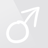# Intro to Measurement System Analysis (MSA) of Continuous Data – Part 1: What and Why?

MSA

Tags:
1.This is the first in a series of articles about MSA. The intent is to share a career’s worth of experience with measurement systems and their application in inspection, process control and continual improvement. Calibration, measurement uncertainty and attribute MSA are outside of the scope of this series and is better left to experts in those fields, of whom we have several in the forum.

What is MSA and why do we need it?

To put it simply, any time we measure a part in order to determine whether it is in specification, whether the process is in a state of statistical control, or whether changing a control factor has a statistically significant effect on a response factor, there is some uncertainty about the true value of the part. Not only does one part vary from the next part, but repeated measurements of the same part will vary.

Why do we see this variation and what causes it? It is important to understand that when we measure a part, we rarely measure the true value of that part. There are many sources of variation that affect the measurement that we obtain using a measurement device, or gage.

These sources of variation include:

• Within part variation in form

• Bias (accuracy)

• Linearity (change over the range of measurement)

• Stability (change over time)

• Repeatability (within operator)

• Reproducibility (between operator)

• Operator * Part interaction
This not only affects the measured value, but also the variation seen as shown below:

X Measured Value = X Actual Value ± X Form ± X Bias ± X Linearity ± X Stability ± X Repeatability ± X Reproducibility ± X Oper.*Part

σ^2Measured = σ^2Actual + σ^2Form + σ^2Bias + σ^2Linearity + σ^2Stability + σ^2Repeatability + σ^2Reproducibility + σ^2 Oper.*Part

The end result of this is threefold:

• Any individual measurement has an uncertainty associated with it. When the specification falls within this zone of uncertainty you have two risks:
• Rejecting a good part (Type I error, Alpha risk)
• Accepting a bad part (Type II error; Beta risk)
• The capability of the process relative to the specifications is degraded by the additional variation added by the measurement system (i.e., your capability is actually better than you realize).
• The number of sample parts required to statistically verify the effect of a change in a control factor on a response factor is increased.
MSA is a methodology for quantify the impact of each of these sources of variation. This allows us to quantify the risk for making Type I and II errors, to understanding the effect of measurement variation on the process capability, as well as the impact on the number of samples required to verify an improvement.
Vicki likes this.

### Recent Reviews

1.Mark Paul
5/5,
Well written as an introduction or a refresher.